• +91 9971497814
• info@interviewmaterial.com

# Chapter 7- Equilibrium Interview Questions Answers

### Related Subjects

Question 1 :
A liquid is in equilibrium with its vapours in a sealed container at a fixed temperature. The volume of the container is suddenly increased,
(i) What is the initial effect of the change on the vapour pressure ?
(ii) How do the rates of evaporation and condensation change initially ?
(iii) What happens when equilibrium is restored finally and what will be the final vapour pressure ?

(i) On increasing the volume of the container, the vapour pressure will initially decrease because the same amount of vapours are now distributed over a larger space.
(ii) On increasing the volume of the container, the rate of evaporation will increase initially because now more space is available. Since the amount of the vapours per unit volume decrease on increasing the volume, therefore, the rate of condensation will decrease initially.
(iii) Finally, equilibrium will be restored when the rates of the forward and backward processes become equal. However, the vapour pressure will remain unchanged because it depends upon the temperature and not upon the volume of the container.

Question 2 :

What is Kc for the following reaction in astate of equilibrium :
2SO2(g)+ O2(g)  2SO3(g) ?
Given : [SO2] = 0.6 M ; [O2]= 0.82 M ; and [SO3] = 1.90 M

Answer 2 :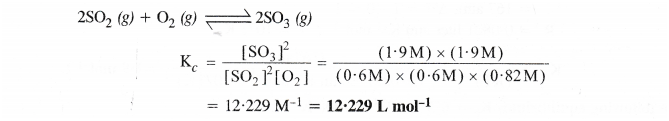Question 3 :

At a certain temperature and total pressureof 10Pa, iodine vapour contains 40% by volume ofI atoms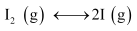Calculate Kpfor the equilibrium.

Partialpressure of I atoms,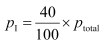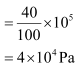Partial pressure of I2 molecules,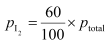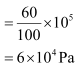Now,for the given reaction,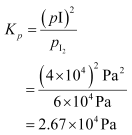Question 4 :

Write the expression for the equilibriumconstant, Kc for each of the following

reactions:

(i)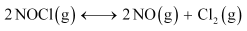(ii)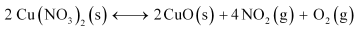(iii)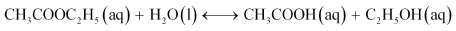(iv)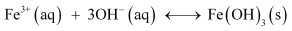(v)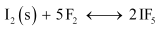Answer 4 :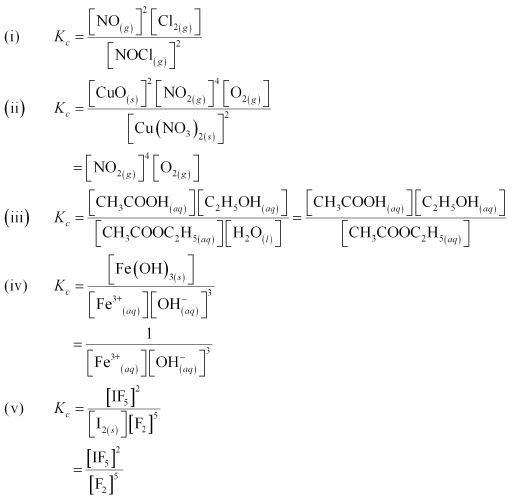Question 5 :

Find out the value of Kc for each of thefollowing equilibria from the value of Kp: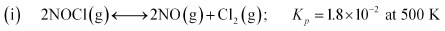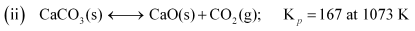The relation between Kp and Kc is given as:

Kp = Kc (RT)Δn

(a) Here,

Δn = 3 – 2 = 1

R = 0.0831 barLmol–1K–1

T = 500 K

Kp = 1.8 × 10–2

Now,

Kp = Kc (RTΔn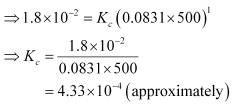(b) Here,

Δn = 2 – 1 = 1

= 0.0831 barLmol–1K–1

= 1073 K

Kp= 167

Now,

Kp = Kc (RTΔn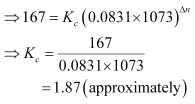Question 6 : For the following equilibrium,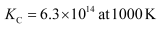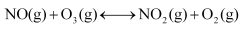Both the forward and reverse reactions inthe equilibrium are elementary bimolecular reactions. What is Kc, for the reversereaction?

Answer 6 : It is given that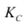for the forward reaction is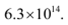Then,forthe reverse reaction will be,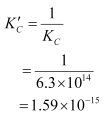Question 7 :

Explainwhy pure liquids and solids can be ignored while writing the equilibriumconstant expression?

Fora pure substance (both solids and liquids),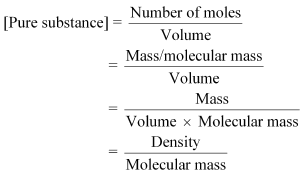Now,the molecular mass and density (at a particular temperature) of a puresubstance is always fixed and is accounted for in the equilibrium constant.Therefore, the values of pure substances are not mentioned in the equilibriumconstant expression.

Question 8 :

Reaction between N2 and O2 takes place asfollows: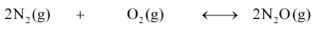If a mixture of 0.482 mol of N2 and 0.933 mol of O2 is placed in a 10 Lreaction vessel and allowed to form N2O at a temperature forwhich Kc = 2.0 × 10–37, determine thecomposition of equilibrium mixture.

Let the concentration of N2O at equilibrium be x.

Thegiven reaction is: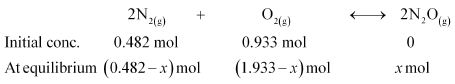Therefore,at equilibrium, in the 10 L vessel: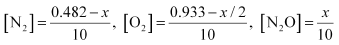The value of equilibrium constant i.e.,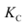= 2.0 × 10–37 is very small.Therefore, the amount of N2 and O2 reacted is also verysmall. Thus, x can be neglected from the expressions of molarconcentrations of N2 and O2.

Then,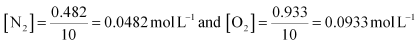Now,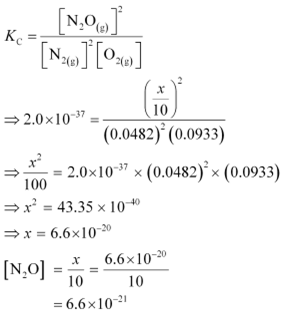Question 9 :

Nitric oxide reacts with Br2 and gives nitrosylbromide as per reaction given below: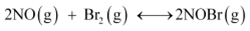When 0.087 mol of NO and 0.0437 mol of Br2 are mixed in aclosed container at constant temperature, 0.0518 mol of NOBr is obtained atequilibrium. Calculate equilibrium amount of NO and Br2.

Thegiven reaction is: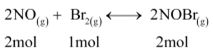Now,2 mol of NOBr are formed from 2 mol of NO. Therefore, 0.0518 mol of NOBr areformed from 0.0518 mol of NO.

Again,2 mol of NOBr are formed from 1 mol of Br.

Therefore,0.0518 mol of NOBr are formed from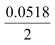molof Br, or

0.0259mol of NO.

Theamount of NO and Br present initially is as follows:

[NO] = 0.087 mol [Br2] = 0.0437 mol

Therefore,the amount of NO present at equilibrium is:

[NO]= 0.087 – 0.0518

=0.0352 mol

And,the amount of Br present at equilibrium is:

[Br2] = 0.0437 – 0.0259

=0.0178 mol

Question 10 :

At 450 K, Kp= 2.0 × 1010/bar for the givenreaction at equilibrium.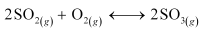What is Kat this temperature?

Forthe given reaction,

Δn = 2 – 3 = – 1

T = 450 K

R = 0.0831 bar L bar K–1 mol–1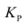= 2.0 × 1010 bar –1

Weknow that,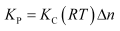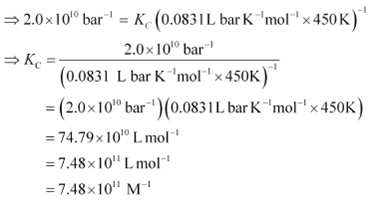Todays Deals### Chapter 7- Equilibrium Contributorskrishan

Name:
Email:

# Latest News# 9000 interview questions in different categories